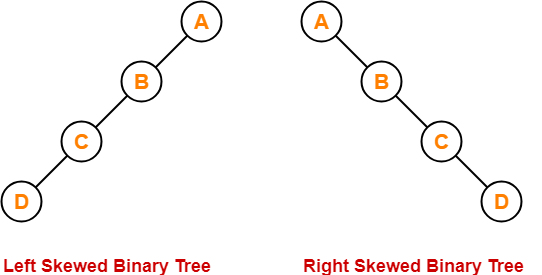154 views

What is the time complexity for insertion in binary tree in worst case?

1. O(1)
2. O(log n)
3. O(n)
4. O(n log n)
| 154 views
+2
In the Worst case, it may grow left skew binary tree or right skew binary tree.

So, time complexity will be $O(n)$

+1 voteFor inserting element as the left child of D(either in a left-skewed binary tree or right skewed binary tree), we have to traverse all elements(i.e. A, B, C, D). Therefore, insertion in the binary tree has worst-case complexity of O(n).

+1 vote
1
2